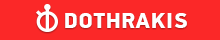GMAT Question of the Day - Daily to your Mailbox; hard ones only

 It is currently 26 Jun 2019, 03:24GMAT Club Daily Prep

Thank you for using the timer - this advanced tool can estimate your performance and suggest more practice questions. We have subscribed you to Daily Prep Questions via email.

Customized
for You

we will pick new questions that match your level based on your Timer History

Track

every week, we’ll send you an estimated GMAT score based on your performance

Practice
Pays

we will pick new questions that match your level based on your Timer History

Not interested in getting valuable practice questions and articles delivered to your email? No problem, unsubscribe here.If the average (arithmetic mean) of 10, 20, 30, 40, and x is 60, what

Author Message
TAGS:

Hide Tags

Math ExpertV
Joined: 02 Sep 2009
Posts: 55802
If the average (arithmetic mean) of 10, 20, 30, 40, and x is 60, what  [#permalink]

Show Tags00:00

Difficulty:5% (low)

Question Stats:94% (00:36) correct6% (00:38) wrongbased on 35 sessions

HideShow timer StatisticsIf the average (arithmetic mean) of 10, 20, 30, 40, and x is 60, what is the value of x?

A. 50
B. 60
C. 100
D. 150
E. 200

_________________
CEOP
Joined: 18 Aug 2017
Posts: 3935
Location: India
Concentration: Sustainability, Marketing
GPA: 4
WE: Marketing (Energy and Utilities)
Re: If the average (arithmetic mean) of 10, 20, 30, 40, and x is 60, what  [#permalink]

Show Tags

Bunuel wrote:
If the average (arithmetic mean) of 10, 20, 30, 40, and x is 60, what is the value of x?

A. 50
B. 60
C. 100
D. 150
E. 200

10+20+30+40+x=60*5
x= 200 option E
_________________
If you liked my solution then please give Kudos. Kudos encourage active discussions.Re: If the average (arithmetic mean) of 10, 20, 30, 40, and x is 60, what   [#permalink] 26 Nov 2018, 23:14
Display posts from previous: Sort by

If the average (arithmetic mean) of 10, 20, 30, 40, and x is 60, what